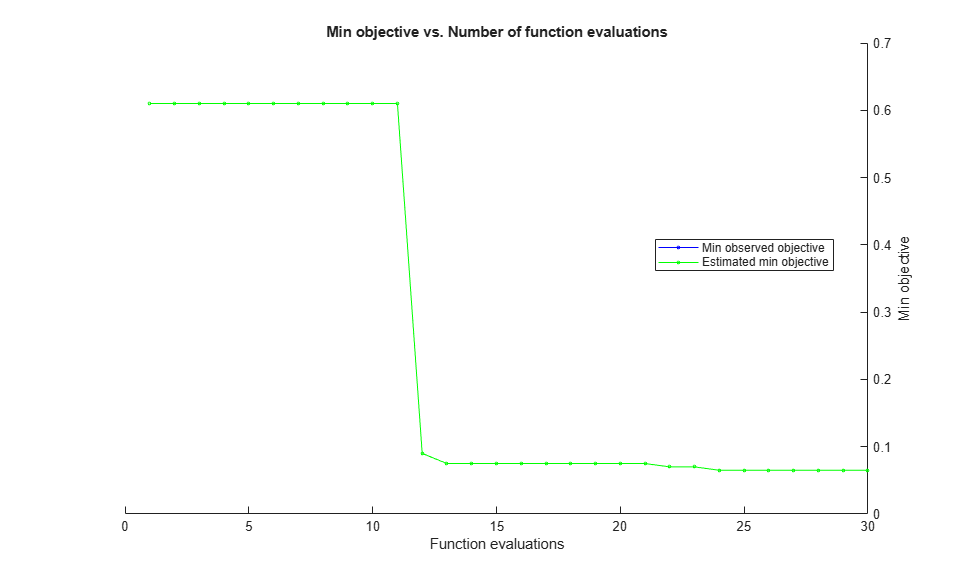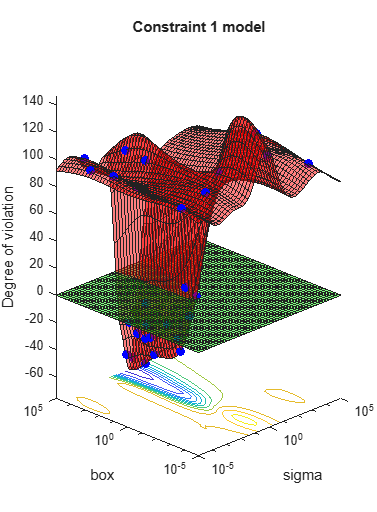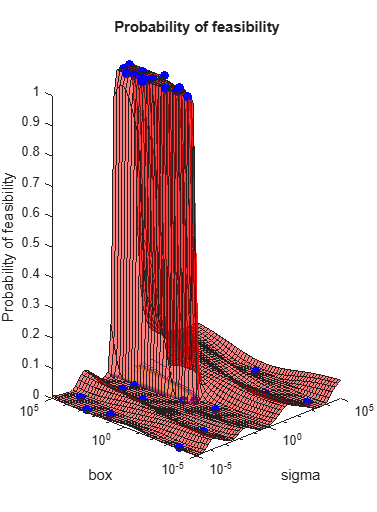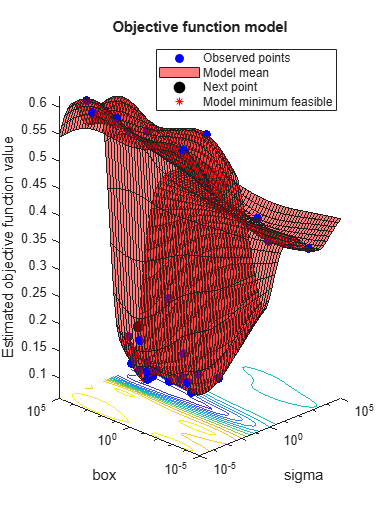# predictConstraints

Predict coupled constraint violations at a set of points

## Syntax

``ConstraintViolations = predictConstraints(results,XTable)``
``````[ConstraintViolations,sigma] = predictConstraints(results,XTable)``````

## Description

example

````ConstraintViolations = predictConstraints(results,XTable)` returns the coupled constraint function violations at the points in `XTable`.```
``````[ConstraintViolations,sigma] = predictConstraints(results,XTable)``` also returns the standard deviations of the coupled constraint functions.```

## Examples

collapse all

This example shows how to predict the coupled constraints of an optimized SVM model. For details of this model, see Optimize Cross-Validated Classifier Using bayesopt.

```rng default grnpop = mvnrnd([1,0],eye(2),10); redpop = mvnrnd([0,1],eye(2),10); redpts = zeros(100,2); grnpts = redpts; for i = 1:100 grnpts(i,:) = mvnrnd(grnpop(randi(10),:),eye(2)*0.02); redpts(i,:) = mvnrnd(redpop(randi(10),:),eye(2)*0.02); end cdata = [grnpts;redpts]; grp = ones(200,1); grp(101:200) = -1; c = cvpartition(200,'KFold',10); sigma = optimizableVariable('sigma',[1e-5,1e5],'Transform','log'); box = optimizableVariable('box',[1e-5,1e5],'Transform','log'); ```

The objective function is the cross-validation loss of the SVM model for the partition `c`. The coupled constraint is the number of support vectors in the model minus 100. The model has 200 data points, so the coupled constraint values range from -100 to 100. Positive values mean the constraint is not satisfied.

```function [objective,constraint] = mysvmfun(x,cdata,grp,c) SVMModel = fitcsvm(cdata,grp,'KernelFunction','rbf',... 'BoxConstraint',x.box,... 'KernelScale',x.sigma); cvModel = crossval(SVMModel,'CVPartition',c); objective = kfoldLoss(cvModel); constraint = sum(SVMModel.IsSupportVector)-100.5; ```

Call the optimizer using this function and its one coupled constraint.

```fun = @(x)mysvmfun(x,cdata,grp,c); results = bayesopt(fun,[sigma,box],'IsObjectiveDeterministic',true,... 'NumCoupledConstraints',1,'PlotFcn',... {@plotMinObjective,@plotConstraintModels,@plotObjectiveModel},... 'AcquisitionFunctionName','expected-improvement-plus','Verbose',0); ```The constraint model plot shows that most parameters in the range are infeasible, and are feasible only for relatively high values of the `box` parameter and a small range of the `sigma` parameter. Predict the coupled constraint values for several values of the control variables `box` and `sigma`.

```sigma = logspace(-2,2,11)'; box = logspace(0,5,11)'; XTable = table(sigma,box); cons = predictConstraints(results,XTable); [XTable,table(cons)] ```
```ans = 11x3 table sigma box cons ________ ______ _______ 0.01 1 99.443 0.025119 3.1623 106.49 0.063096 10 94.468 0.15849 31.623 25.134 0.39811 100 -38.732 1 316.23 -55.156 2.5119 1000 -34.181 6.3096 3162.3 5.0153 15.849 10000 39.465 39.811 31623 60.9 100 1e+05 71.906 ```

## Input Arguments

collapse all

Bayesian optimization results, specified as a `BayesianOptimization` object.

Prediction points, specified as a table with D columns, where D is the number of variables in the problem. The function performs its predictions on these points.

Data Types: `table`

## Output Arguments

collapse all

Constraint violations, returned as an `N`-by-`K` matrix, where there are `N` rows in `XTable` and `K` coupled constraints. The constraint violations are the posterior means of the Gaussian process model of the coupled constraints at the points in `XTable`.

Constraint standard deviations, returned as an `N`-by-`K` matrix, where there are `N` rows in `XTable` and `K` coupled constraints. The standard deviations represent those of the posterior distribution at the points in `XTable`.

## Version History

Introduced in R2016b